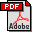The Impact of Two-dimensional Block Cyclic Distribution in Accuracy and Performance of Parallel Linear Algebra Subroutines
Mariana Kolberg (ULBRA/ PUCRS)
Björn Rocker (Karlsruhe Institute of Technology (KIT))
Vincent Heuveline (Karlsruhe Institute of Technology (KIT))
Abstract:
In parallel computing the data distribution may have a significant impact in the application performance and accuracy. These effects can be observed using the parallel matrix-vector multiplication routine from PBLAS with different grid configuration for the data distributions. Matrix-vector multiplication is an especially important operation once it is widely used in numerical simulation (e.g., iterative solvers for linear systems of equations). This paper presents a mathematical background of error propagation in elementary operations and proposes benchmarks to show how different grids configuration on two dimensional cyclic block distribution impacts on accuracy and performance using parallel matrix-vector operations. The experimental results validate the theoretical findings.
Keywords:
Parallel and Distributed Computing, Numerical Algorithms for CS&E, Performance AnalysisDownload the fullpaper